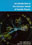© CAMBRIDGE UNIVERSITY PRESS 1998

### 1. THE ARTICLE PHYSICIST'S VIEW OF NATURE

1.1 Introduction

It is more than a century since the discovery by J.J. Thomson of the electron. The electron is still thought to be a structureless point particle, and one of the elementary particles of Nature. Other particles which were subsequently discovered and at first thought to be elementary, like the proton and the neutron, have since been found to have a complex structure.

What then are the ultimate constituents of matter? How are they categorised? How do they interact with each other? What, indeed, should we ask of a mathematical theory of elementary particles? Since the discovery of the electron, and more particularly in the last fifty years, there has been an immense amount of experimental and theoretical effort to determine answers to these questions. The present Standard Model of particle physics stems from that effort.

The Standard Model asserts that the material in the universe is made up of elementary fermions interacting through fields, of which they are the sources. The particles associated with the interaction fields are bosons.

Four types of interaction field, set out in Table 1.1, have been distinguished in Nature. On the scales of particle physics, gravitational forces are insignificant. The Standard Model excludes from consideration the gravitational field. The quanta of the electromagnetic interaction field between electrically charged fermions are the massless photons. The quanta of the weak interaction fields between fermions are the charged W+ and W- bosons and the neutral Z boson, discovered at CERN in 1983. Since these carry mass, the weak interaction is short ranged: by the uncertainty principle, a particle of mass M can exist as part of an intermediate state for a time/ Mc2, and in this time the particle can travel a distance no greater thanc / Mc2. Since MW80 GeV / c2 and MZ90 GeV / c2, the weak interaction has a range10-3 fm.

 Interaction field Boson Spin Gravitational field ``Gravitons'' postulated 2 Weak field W+, W-, Z particles 1 Electromagnetic field Photons 1 Strong field ``Gluons'' postulated 1

The quanta of the strong interaction field, the gluons, have zero mass, and, like photons might be expected to have infinite range. However, unlike the electromagnetic field, the gluon fields are confining, a property we shall be discussing at length in the later chapters of this book.

The elementary fermions of the Standard Model are of two types: leptons and quarks. All have spin 1/2, in units of, and in isolation would be described by the Dirac equation, which we discuss in Chapters 5, 6 and 7. Leptons interact only through the electromagnetic interaction (if they are charged) and the weak interaction. Quarks interact through the electromagnetic and weak interactions and also through the strong interaction.